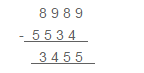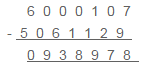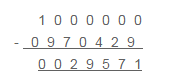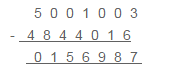×#### Thank you for registering.

One of our academic counsellors will contact you within 1 working day.

Click to Chat

1800-1023-196

+91-120-4616500

CART 0

• 0

MY CART (5)

Use Coupon: CART20 and get 20% off on all online Study Material

ITEM
DETAILS
MRP
DISCOUNT
FINAL PRICE
Total Price: Rs.

There are no items in this cart.
Continue Shopping• Complete JEE Main/Advanced Course and Test Series
• OFFERED PRICE: Rs. 15,900
• View Details

```Chapter 4: Operations on Whole Numbers – Exercise 4.2

Question: 1

A magic square is an array of numbers having the same number of rows and columns and the sum of numbers in each row, column or diagonal being the same. Fill in the blank cells of the following magic squares:

(i)

8
13

12

11

(ii)

22

6
13
20

10
12
19

9
11
18
25

15
17
24
26

16

7
14

Solution:

(i) It can be seen that diagonally, 13 + 12 + 11 = 36.

Thus,

Number in the first cell of the first row = 36 – (8 + 13) = 15

Number in the first cell of the second row = 36 – (15 + 11) = 10

Number in the third cell of the second row = 36 – (10 + 12) = 14

Number in the second cell of the third row = 36 – (8 + 12) = 16

Number in the third cell of the third row = 36 – (11 + 16) = 9

15
8
13

10
12
141

11
16
9

(ii) It can be seen that diagonally, 20 + 19 + 18 + 17 + 16 = 90.

Thus,

Number in the second cell of the first row = 90 – (22 + 6 + 13 + 20) = 29

Number in the first cell of the second row = 90 – (22 + 9 + 15 + 16) = 28

Number in the fifth cell of the second row = 90 – (28 + 10 + 12 + 19) = 21

Number in the fifth cell of the third row = 90 – (9 + 11 + 18 + 25) = 27

Number in the fifth cell of the fourth row = 90 – (15 + 17 + 24 + 26) = 8

Number in the second cell of the fifth row = 90 – (29 + 10 + 11 + 17) = 23

Number in the third cell of the fifth row = 90 – (6 + 12 + 18 + 24) = 30

22
29
6
13
20

28
10
12
19
21

9
11
18
25
27

15
17
24
26
8

16
23
30
7
14

Question: 2

Perform the following subtractions and check your results by performing corresponding additions:

Solution:

(i) 57839 – 2983 = 54856

Verification: 54856 + 2983 = 57839

(ii) 92507 – 10879 = 81628

Verification: 81628 + 10879 = 92507

(iii) 400000 – 98798 = 301202

Verification: 301202 + 98798 = 400000

(iv) 5050501 – 969696 = 4080805

Verification: 4080805 + 969696 = 5050501

(v) 200000 – 97531 = 102469

Verification: 102469 + 97531 = 200000

(vi) 3030301 – 868686 = 2161615

Verification: 2161615 + 868686 = 3030301

Question: 3

Replace each * by the correct digit in each of the following:

Solution:Here, we can we see that in the units digit, 6 – * = 7, which means that the value of * is 9, as 1 gets carried from 7 at tens place to 6 at unit place and 6 at unit digit becomes 16 then 16 – 9 = 7.

Now, when 7 gives 1 to 6, it becomes 6, so 6 – 3 = 3.

Also, it can be easily deduced that in (8 – * = 6 ), the value of * is 2.(ii) Here, it is clear that in the units place, 9 – 4 = 5;

And in the tens place,

8 – 3 = 5.

We can now easily find out the other missing blanks by subtracting 3455 from 8989. Addend (difference) = 3455(iii)Here, in the units digit, 17 – 8 = 9; in the tens digit, 9 – 7 = 2;

in the hundreds place, 10 – 9 = 1;

and in the thousands place, 9 – 8 = 1.

So, in order to get the addend, we will subtract 5061129 from 6000107.(iv)In the units place, 10 -1 = 9;

Also, in the lakhs place, 9 -0 = 9;

So, in order to get the addend, we will subtract 970429 from 1000000.(v)Here, in the units digit, 13 – 7 = 6;

in the tens digit, 9 – 8 = 1;

in the hundreds place, 9 – 9 = 0;

and in the thousands place, 10 – 6 = 4.

So, in order to get the addend, we will subtract 4844016 from 5001003.(vi)It is clear from the units place that 11 – 9 = 2.

To get the other addend, we will subtract 54322 from 111111.Thus, the other addend is 56789.Question: 4

What is the difference between the largest number of five digits and smallest number of six digits?

Solution:

The largest five – digit number is 99999.

The smallest six – digit number is 100000.

Therefore, difference between them = 100000 – 99999 = 1

Question: 5

Find the difference between the largest number of 4 digits and the smallest number of 6 digits.

Solution:

The largest four – digit number is 9999.

The smallest seven – digit number is 1000000.

Therefore, difference between them = 1000000 – 9999 = 990001

Question: 6

Rohit deposited Rs 125000 in his savings bank account. Later he withdrew Rs 35425 from it. How much money was left in his account?

Solution:

Money deposited by Rohit = Rs 125000

Money withdrawn by Rohit = Rs 35425

Therefore, money left in the account = Rs (125000 – 35425) = Rs 89575

Question: 7

The population of a town is 96209. If the number of men is 29642 and that of women is 29167, determine the number of children.

Solution:

Total population of the town = 96209

Number of men = 29642

Number of women = 29167

Sum of men and women = (29642 + 29167) = 58809

Therefore, Number of children in the town = ( Total population ) – ( Sum of men and women )

= 96209 – 58809 = 37400

Question: 8

The digits of 6 and 9 of the number 36490 are interchanged. Find the difference between the original number and the new number.

Solution:

Original number = 39460

New number = – 36490

Difference = 39460 – 36490 = 2970

Question: 9

The population of a town was 59000. In one year it was increased by 4563 due to new births. However, 9218 persons died or left the town during the year. What was the population at the end of the year?

Solution:

Population of the town = 59000

Increase in the population = 4536

Decrease in the population = 9218

New population = 59000 + 4536 – 9218 = 54318
```### Course Features

• 728 Video Lectures
• Revision Notes
• Previous Year Papers
• Mind Map
• Study Planner
• NCERT Solutions
• Discussion Forum
• Test paper with Video Solution